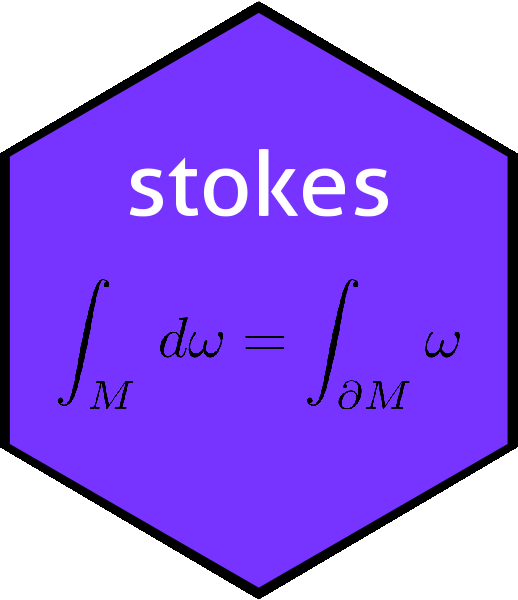# The dovs() function in the stokes package: the dimension of the underlying vector spacedovs
## function (K)
## {
##     if (is.zero(K) || is.scalar(K)) {
##         return(0)
##     }
##     else {
##         return(max(index(K)))
##     }
## }
## <bytecode: 0x559b66641c28>
## <environment: namespace:stokes>

Function dovs() returns the dimensionality of the underlying vector space of a $$k$$-form. Recall that a $$k$$-form is an alternating linear map from $$V^k$$ to $$\mathbb{R}$$, where $$V=\mathbb{R}^n$$. Function dovs() returns $$n$$ [compare arity(), which returns $$k$$]. As seen above, the function is very simple, essentially being max(index(K)), but its use is not entirely straightforward in the context of stokes idiom. Consider the following:

set.seed(0)
a <- rform(n=4,k=2)
a
## An alternating linear map from V^2 to R with V=R^4:
##          val
##  2 4  =    9
##  1 4  =    8
##  2 3  =    1
##  1 3  =   -3
##  3 4  =   -2
##  1 2  =    2

Now object a is notionally a map from $$\left(\mathbb{R}^4\right)^2$$ to $$\mathbb{R}$$:

f <- as.function(a)
(M <- matrix(1:8,4,2))
##      [,1] [,2]
## [1,]    1    5
## [2,]    2    6
## [3,]    3    7
## [4,]    4    8
f(M)
##  -148

However, a can equally be considered to be a map from $$\left(\mathbb{R}^5\right)^2$$ to $$\mathbb{R}$$:

f <- as.function(a)
(M <- matrix(c(1,2,3,4,1454,5,6,7,8,-9564),ncol=2))  # row 5 large numbers
##      [,1]  [,2]
## [1,]    1     5
## [2,]    2     6
## [3,]    3     7
## [4,]    4     8
## [5,] 1454 -9564
f(M)
##  -148

If we view $$a$$ [or indeed f()] in this way, that is $$a\colon\left(\mathbb{R}^5\right)^2\longrightarrow\mathbb{R}$$, we observe that row 5 is ignored: $$e_5=\left(0,0,0,0,1\right)^T$$ maps to zero in the sense that $$f(e_5,\mathbf{v})=f(\mathbf{v},e_5)=0$$, for any $$\mathbf{v}\in\mathbb{R}^5$$.

(M <- cbind(c(0,0,0,0,1),runif(5)))
##      [,1]      [,2]
## [1,]    0 0.3800352
## [2,]    0 0.7774452
## [3,]    0 0.9347052
## [4,]    0 0.2121425
## [5,]    1 0.6516738
f(M)
##  0

(above we see that rows 1-4 of M are ignored because of the zero in column 1; row 5 is ignored because the index of a does not include the number 5). Because a is alternating, we could have put $$e_5$$ in the second column with the same result. Alternatively we see that the $$k$$-form a, evaluated with $$e_5$$ as one of its arguments, returns zero because the index matrix of a does not include the number 5. Most of the time, this kind of consideration does not matter. However, consider this:

dx
## An alternating linear map from V^1 to R with V=R^1:
##        val
##  1  =    1

Now, we know that dx is supposed to be a map from $$\left(\mathbb{R}^3\right)^1$$ to $$\mathbb{R}$$; but:

dovs(dx)
##  1

So according to stokes, $$\operatorname{dx}\colon\left(\mathbb{R}^1\right)^1\longrightarrow\mathbb{R}$$. This does not really matter numerically, until we consider the Hodge star operator. We know that $$\star\operatorname{dx}=\operatorname{dy}\wedge\operatorname{dz}$$, but

hodge(dx)
##  1

Above we see the package giving, correctly, that the Hodge star of $$\operatorname{dx}$$ is the zero-dimensional volume element (otherwise known as “1”). To get the answer appropriate if $$\operatorname{dx}$$ is considered as a map from $$\left(\mathbb{R}^3\right)^1$$ to $$\mathbb{R}$$ [that is, $$\operatorname{dx}\colon\left(\mathbb{R}^3\right)^1\longrightarrow\mathbb{R}$$], we need to specify dovs explicitly:

hodge(dx,3)
## An alternating linear map from V^2 to R with V=R^3:
##          val
##  2 3  =    1

Actually this looks a lot better with a more appropriate print method:

options(kform_symbolic_print="dx")
hodge(dx,3)
## An alternating linear map from V^2 to R with V=R^3:
##  + dy^dz